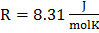### Sample Problem

Consider the following reaction:Is the forward reaction spontaneous when the concentrations of [N2], [H2], and [NH3] are 0.500 M, 0.750 M, and 1.25 M respectively at 375 K?#### Solution

The change in free energy is related to the standard free energy as shown in the following equationwhere R is the gas constant, T is the absolute temperature, and Q is the reaction quotientBecause ΔG is negative, the forward reaction is spontaneous.